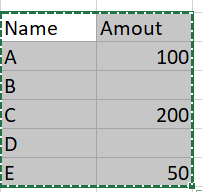# How to handle null values

Hi,

I have a Data table that is having empty value and integer value. I want to sum the column but as i have empty values also in the column it is giving me error

DataTableBelow is my query

dt.AsEnumerable.Sum(Function(a)Convert.ToDouble(a(“Amount”).ToString))

it is working fine when we dont have null value, could you please suggest how to handle null value in the query

Thank you

can you try this

Dt_Data.AsEnumerable.Sum(Function(su) If(Int32.TryParse(su(IndexOrColumnName).ToString.Trim, New Int32),Int32.Parse(su(IndexOrColumnName).ToString.Trim),0))

Thanks

1 Like

Hi,

FYI, another solution:

``````dt.AsEnumerable.Where(Function(r) Double.TryParse(r("Amount").ToString,New Double)).Sum(function(r) Double.Parse(r("Amount").ToString))
``````

Regards,

1 Like

Yes, it worked… Thank you

Thank you, for the solution

did it work @RENU_KHALKHO

This topic was automatically closed 3 days after the last reply. New replies are no longer allowed.